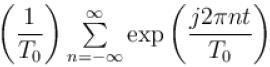Courses

# Test: Fourier Series- 1

## 10 Questions MCQ Test Topic wise Tests for IIT JAM Physics | Test: Fourier Series- 1

Description
This mock test of Test: Fourier Series- 1 for IIT JAM helps you for every IIT JAM entrance exam. This contains 10 Multiple Choice Questions for IIT JAM Test: Fourier Series- 1 (mcq) to study with solutions a complete question bank. The solved questions answers in this Test: Fourier Series- 1 quiz give you a good mix of easy questions and tough questions. IIT JAM students definitely take this Test: Fourier Series- 1 exercise for a better result in the exam. You can find other Test: Fourier Series- 1 extra questions, long questions & short questions for IIT JAM on EduRev as well by searching above.
QUESTION: 1

### The Fourier series expansion of a real periodic signal with fundamental frequency f0 is given by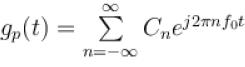It is given that C3 = 3 + 5j then C-3 is Select one:

Solution:

Given  C3 = 3 + 5j
We know that for real periodic signal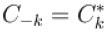So,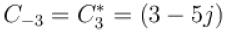The correct answer is: 3 – 5j

QUESTION: 2

### The Fourier series of an odd periodic function, contains only Select one:

Solution:

If periodic function is odd the dc term a0 = 0 and also cosine terms (even symmetry)
It contains only sine terms.
The correct answer is: sine terms

QUESTION: 3

### The trigonometric Fourier series of an even function of time does not have Select one:

Solution:

For periodic even function, the trigonometric Fourier series does not contain the sine terms (odd functions.)
It has dc term and cosine terms of all harmonic.
The correct answer is: Sine terms

QUESTION: 4

Trigonometric Fourier series of a periodic time function can have only
Select one:

Solution: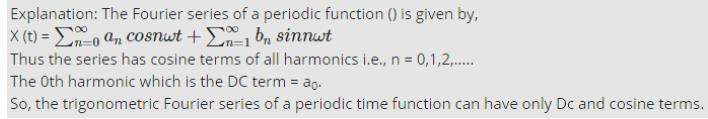QUESTION: 5

Which of the following cannot be the Fourier series expansion of a periodic signals?
Select one:

Solution:

→  x(t) = 2cos t + 3cos t is periodic signal with fundamental frequency ω = 1.
→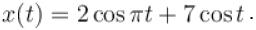The frequency of first term  frequency of 2nd term is ω2 = 1.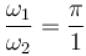is not the rational number
So, x(t) is a periodic or not periodic.
→ x(t) = cos t + 0.5 is a periodic function with
→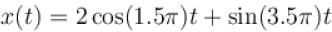first term has frequency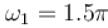2nd term has frequency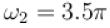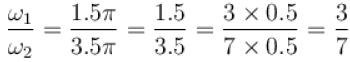So about ratio is rational number x(t) is a periodic signal, with fundamentalfrequency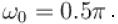Since function in (b) is non periodic. So does not satisfy Dirichlet conditionand cannot be expanded in Fourier series.
The correct answer is: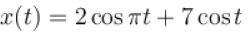QUESTION: 6

A periodic signal x(t) of period T0 is given by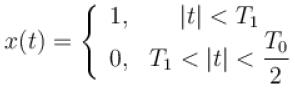The dc component of x(t) is
Select one:

Solution:

Solution :- The dc component of x(t) is :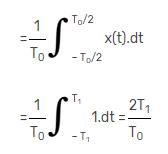QUESTION: 7

The trigonometric Fourier series of an even function does not have the
Select one:

Solution:

The trigonometric Fourier series of an even function has cosine terms which are even functions.
It has dc term if its average value is finite and no dc term if average value is zero.
So it does not have sine terms.
The correct answer is: Sine terms

QUESTION: 8

Choose the function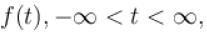for which a Fourier series cannot be defined
Select one:

Solution:

→  3sin(25t) is periodic ω = 25.
→ 4cos(20t + 3) + 2sin(710t) sum of two periodic function is also periodic function
→ e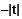sin 25t Due to decaying exponential decaying function it is not periodic.
So Fourier series cannot be defined for it.
→ Constant, Fourier series exists.
Fourier series can’t be defined for option (c).
The correct answer is: exp(–|t|) sin(25t)

QUESTION: 9

The Fourier series of a real periodic function has only
P. Cosine terms if it is even
Q. Sine terms if it is even
R. Cosine terms if it is odd
S. Sine terms if it is odd
Which of the above statement is correct?
Select one:

Solution:

The Fourier series for a real periodic function has cosine terms if it is even and sine terms if it is odd.
The correct answer is: P and S

QUESTION: 10

The Fourier series representation of an impulse train denoted by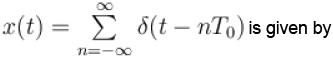Select one:

Solution: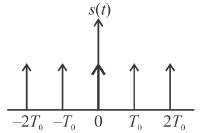The given impulse train s(t) with strength of each impulse as 1 is a periodic function with period T0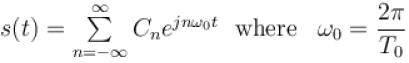where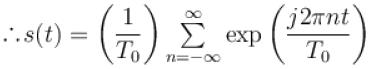The correct answer is: